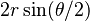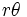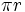# Metrics on the circle

(diff) ← Older revision | Latest revision (diff) | Newer revision → (diff)
Under the standard embedding of the circle inside Euclidean space, we can give it the subspace metric. If the circle is embedded as having radius$r$, the subspace metric gives distance$2r$ to diametrically opposite points, and in general, for points separated by an angle of$\theta$, it gives the distance$2r \sin (\theta/2)$.
The arclength metric is the most natural metric on the circle. It arises as the metric from the Riemannian metric on the circle, and is a totally geodesic metric. The distance between two points is the length of the arc between them. Thus, if the circle is thought of as having radius$r$, the arclength metric gives a distance of$r\theta$ for points with an angular separation of$\theta$. Diametrically opposite points are separated by a distance of$\pi r$.